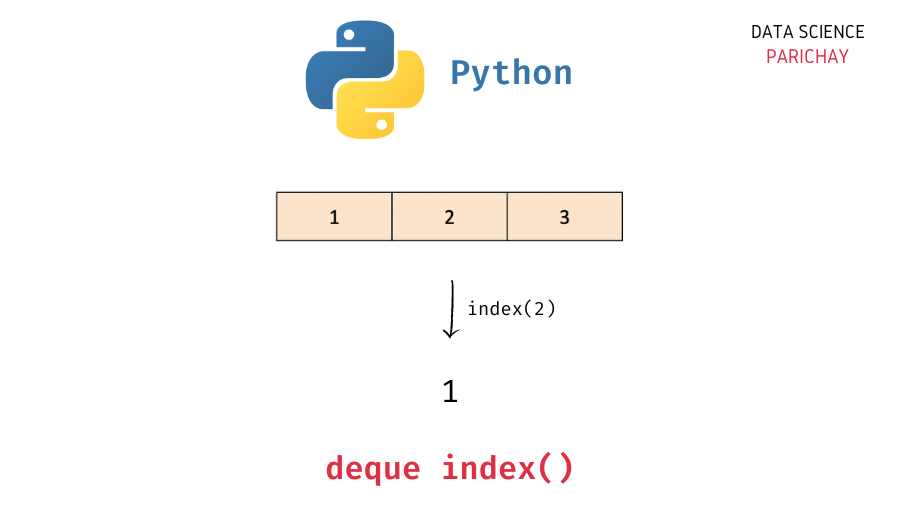# Python – Get Index of a Value in Deque

In this tutorial, we will look at how to get the index of a value in a deque in Python with the help of some examples.

## `deque` in Python

The `collections` module in Python comes with a `deque` class that you can use to implement a stack or queue data structure in Python. Deques support thread-safe, memory efficient appends and pops from either side of the deque with approximately the same O(1) performance in either direction.

In simple terms, you can think of a `deque` in Python as a list-like data structure but with efficient insertion and removal of items from either end (left and right). This makes them very useful when implementing a stack or queue functionality in Python.

## How to get the index of a value in a deque in Python?

The `deque` class comes with a number of built-in functions to help perform common operations (like adding and removing elements).

To get the index of a value in a deque in Python, use the deque `index()` function. Pass the value for which you want the index as an argument. The following is the syntax –

```# index of element "e" in deque "queue"
queue.index(e)```

It returns the index of the first matching value in the deque.
Note that the deque `index()` function also takes optional parameters (for the start index and the stop index) via which you can define the range of indices to look inside of for the given value.

## Examples

Let’s now look at some examples of using the above syntax –

### Example 1 – Get the index of a value in a deque

First, let’s create a deque with some elements.

📚 Data Science Programs By Skill Level

Introductory

Intermediate ⭐⭐⭐

🔎 Find Data Science Programs 👨‍💻 111,889 already enrolled

Disclaimer: Data Science Parichay is reader supported. When you purchase a course through a link on this site, we may earn a small commission at no additional cost to you. Earned commissions help support this website and its team of writers.

```from collections import deque

# create a deque
queue = deque([1, 2, 3])
# print the deque
print(queue)```

Output:

`deque([1, 2, 3])`

Here, we imported the `deque` class from the `collections` module and created a deque object, `queue` with three elements – 1, 2, and 3.

Now, let’s now get the index of the element `2` from the deque created above.

```# index of element 2 in the deque
print(queue.index(2))```

Output:

`1`

Here, we used the deque `index()` function to get the index of the element `2` in the deque `queue`. We get its index as 1.

What happens if there are multiple elements with the same value?

In that case, the deque `index()` function will return the index of the first matching value (inside the given `start` and `stop` range, if `start` and `stop` are not explicitly defined it will look for the value in the entire deque).

Let’s look at an example.

```# create a deque
queue = deque([1, 2, 3, 2, 4])
# index of element 2 in the deque
print(queue.index(2))```

Output:

`1`

Here, the deque `queue` contains the element `2` at two different indices. When we use the deque `index()` function and pass `2`, we get the index of its first occurrence.

You can further customize the search for the value by using optional parameters for the stop and stop indices. These parameters let you define the range of indices in which you want to look for your value.

For example, to get the first index of the element `2` starting from the index 2, pass `2` after the value you’re looking for.

```# create a deque
queue = deque([1, 2, 3, 2, 4])
# get index of first occurrence of element 2 from index 2
print(queue.index(2, 2))```

Output:

`3`

We get the index of the first occurrence of the element `2` starting from index 2.

### Example 2 – Get Index of value not present in the deque

Let’s now try to get the index of an element that is not present in a deque.

```# create a deque
queue = deque([1, 2, 3])
# index of element 4 in the deque
print(queue.index(4))```

Output:

```ValueError                                Traceback (most recent call last)
Input In , in <module>
2 queue = deque([1, 2, 3])
3 # index of element 4 in the deque
----> 4 print(queue.index(4))

ValueError: 4 is not in deque```

Here, we created a deque with elements 1, 2, and 3 and then used the `index()` function to get the index of the element 4 (which is not present in the deque). Since the element is not in the deque, we get a `ValueError`.

You might also be interested in –

•